From a point on the ground, 20 m away from the foot of a vertical tower
Question:

From a point on the ground, 20 m away from the foot of a vertical tower, the angle elevation of the top of the tower is 60°, What is the height of the tower?

Solution:

Let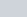be the height of tower is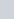meters.Given that: angle of elevation is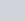and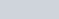meters.

Here we have to find height of tower.

So we use trigonometric ratios.

In a triangle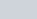,

$\Rightarrow \tan C=\frac{A B}{B C}$

$\Rightarrow \tan 60^{\circ}=\frac{h}{20}\left[\because \tan 60^{\circ}=\sqrt{3}\right]$

$\Rightarrow \sqrt{3}=\frac{h}{20}$

$\Rightarrow h=20 \sqrt{3}$

Hence height of tower is $20 \sqrt{3}$.